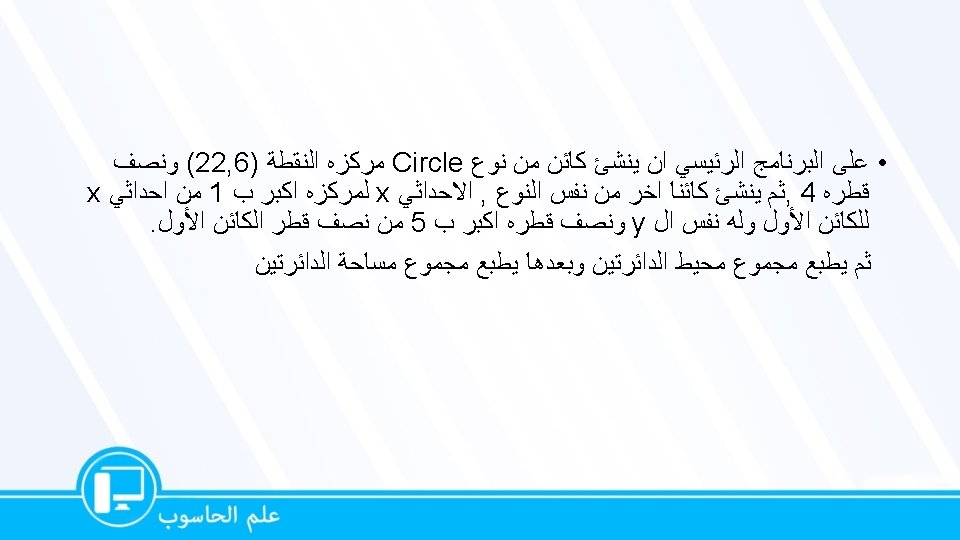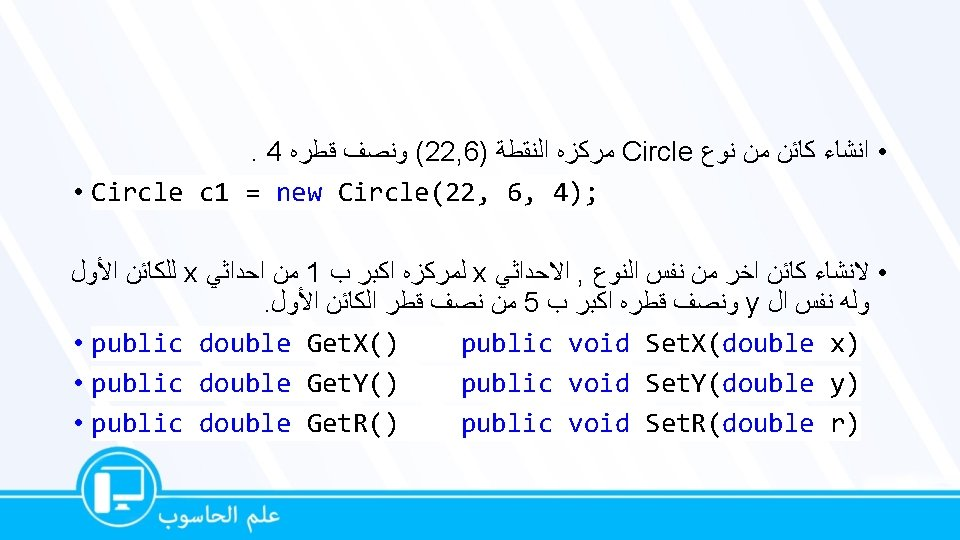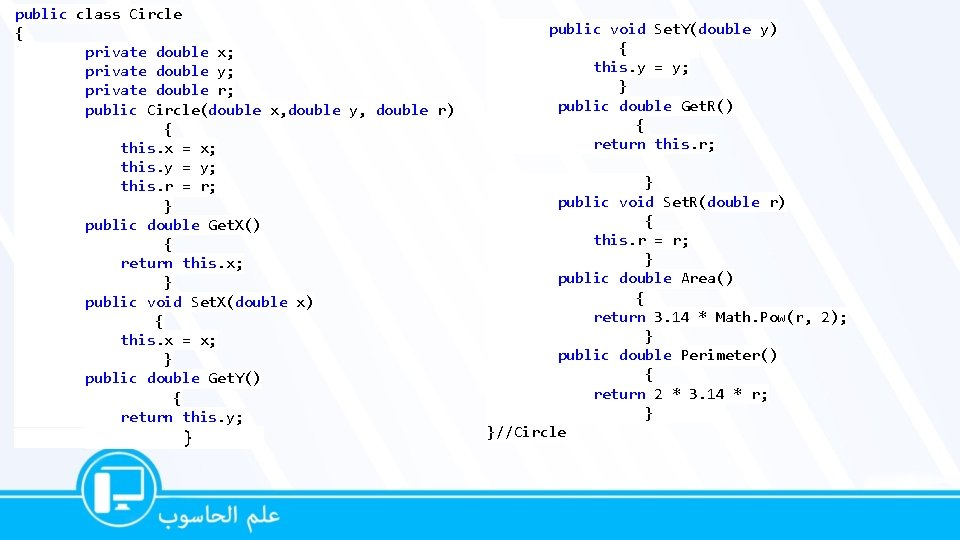# Circle private double x private double y private

• Slides: 8: Circle ﺑﻨﺎﺀ ﺍﻟﻔﺌﺔ private double x; private double y; private double r; – ﺍﻟﻌﻤﻠﻴﺔ ﺍﻟﺒﻨﺎﺋﻴﺔ • public Circle(double x, double y, double r) • { • this. x = x; • this. y = y; • this. r = r; • } • • •public class Circle { private double x; private double y; private double r; public Circle(double x, double y, double r) { this. x = x; this. y = y; this. r = r; } public double Get. X() { return this. x; } public void Set. X(double x) { this. x = x; } public double Get. Y() { return this. y; } public void Set. Y(double y) { this. y = y; } public double Get. R() { return this. r; } public void Set. R(double r) { this. r = r; } public double Area() { return 3. 14 * Math. Pow(r, 2); } public double Perimeter() { return 2 * 3. 14 * r; } }//Circleclass Program { static void Main() { Circle c 1 = new Circle(22, 6, 4); Circle c 2; c 2 = new Circle(c 1. Get. X() + 1, c 1. Get. Y(), c 1. Get. R() + 5); Console. Write. Line(c 1. Perimeter() + c 2. Perimeter()); Console. Write. Line(c 1. Area() + c 2. Area()); } }